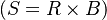# Simulation Models

## Definition

(Monte Carlo) Simulation is a specific mathematical technique whereby multiple scenarios are constructed and analysed via the generation of artificially generated random outcomes.

The model output may be an average over scenarios or a specific quantile.

Simulation is typically employed when the underlying model is too complex to yield explicit Analytic Models or Semi-Analytic Models

## Root and Branch Simulation

In order to form expectations at future timepoints the set of total scenarios S is organized in groups of root and branch scenarios$(S = R \times B)$.

A root scenario is a multiperiod realization of uncertainty factors that starts at the current time t=0 and extends till maturity T. A branch scenario is identical with its root scenario until a specified Risk Horizon but it then potentially branches off. Expectations at the risk horizon for each root scenario are calculated by averaging the branch scenarios

## Issues and Challenges

• Simulation models may be subject to stability issues if limited by amount of available computational time.